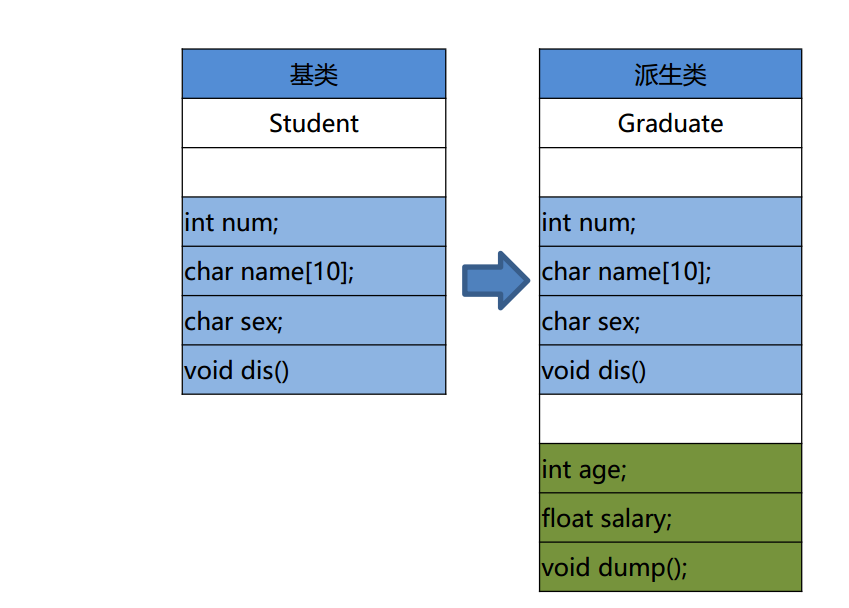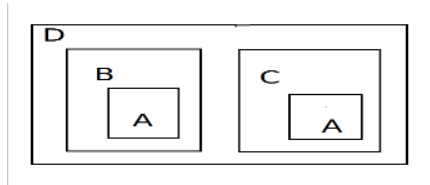# C++之继承(Inheritance)

``````class 派生类名： [继承方式] 基类名
{
派生类成员声明；
};``````

## 1、继承方式

public继承时：父类中的protected是只有被继承的子类和本类的成员函数可以访问。父类中的private成员，子类也无法访问。

1 全盘接收， 除了构造器与析构器。 基类有可能会造成派生类的成员冗余， 所以说基类是需设计的。

2 派生类有了自己的个性， 使派生类有了意义。## 2、构造顺序

``````派生类名::派生类名(总参列表)
:基类名(参数表),内嵌子对象(参数表)
{
派生类新增成员的初始化语句; //也可出现地参数列表中
}``````

## 3、派生类的拷贝构造

``````#include <iostream>
using namespace std;

class A
{
public:
A()
{
cout<<"A()"<<endl;
}

A(const A & anthter)
{
cout<<"A(const A & anthter)"<<endl;
}
};

class C
{
public:
C()
{
cout<<"C()"<<endl;
}
C(const C & another)
{
cout<<"C(const c & another)"<<endl;
}
};

class B:public A
{
public:
B()
{
cout<<"B()"<<endl;
}
B(const B & another)
:A(another),_c(another._c)  //初始化列表调用父类和内嵌子对象的拷贝构造器，要用对象名，不是类名
{ }

C _c;
};

int main()
{
B b;
cout<<"+++++++++++++++"<<endl;
B bb(b);
return 0;
}``````

## 4、派生类的赋值重载

``````#include <iostream>
using namespace std;
class A
{
public:
A()
{
cout<<"A"<<endl;
}
A& operator=(const A &)
{
cout<<" A & operator=(const A &)"<<endl;
}
};
class C
{
public:
C()
{
cout<<"C()"<<endl;
}

C& operator=(const C &)
{
cout<<" C & operator=(const C &)"<<endl;
}
};

class B:public A
{
public:
B & operator=(const B &another)
{
_c = another._c;//实现内嵌子对象的赋值重载，调用子对象的=
A::operator=(another);//实现父类的赋值重载，调用子对象的=
cout<<" B & operator=(const B &)"<<endl;
}
C _c; //内嵌子对象
};
int main()
{
B b1,b2;
cout<<"++++++++++"<<endl;
b1 = b2;
return 0;
}``````

## 5、派生类的友元函数

``````#include <iostream>
using namespace std;

class Student
{
friend ostream &operator<<(ostream & out, Student & stu);//父类友元函数
public:
Student(int i,int j)
:a(i),b(j){}
private:
int a;
int b;
};

ostream &operator<<(ostream & out, Student & stu) //全局友元函数
{
out<<stu.a<<endl;
out<<stu.b<<endl;
}

{
friend ostream &operator<<(ostream & out, Graduate & gra) //子类友元函数
{
cout<<(Student&)gra; //要强制类型转化为(Student&)
cout<<gra.c<<endl;
}
public:
:Student(i,j),c(k){}
private:
int c;
};

int main()
{
cout<<g<<endl;
return 0;
}
``````

## 7、多继承

``派生类名:public 基类名 1， public 基类名 2， ...,protected 基类名 n``

``````派生类名::派生类名(总参列表)
： 基类名 1(参数表 1)， 基类名(参数名 2)....基类名 n(参数名 n)，
内嵌子对象 1(参数表 1),内嵌子对象 2(参数表 2)...内嵌子对象 n(参数表 n)
{
派生类新增成员的初始化语句；
} ``````

### 三角问题

``````void dis()
{
cout<<X::_data<<endl;
cout<<Y::_data<<endl;
} ``````

### 虚继承——四角问题

​ 三角关系中需要解决的问题有两类：

• 数据冗余问题，
• 访问不方便的问题。

• 提取公共成员构成祖父类， 即虚基类，
• 各父类虚继承虚基类### 虚基类

``class 派生类名:virtual 继承方式 虚基类``

### 初始化顺序

``class SofaBed:public Sofa, public Bed``

Last modification：October 6th, 2019 at 02:15 pm